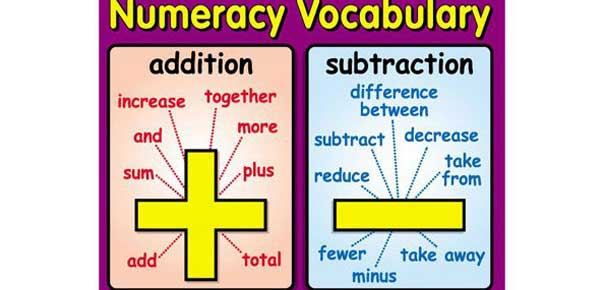# Basic Math Terms About Math Vocabulary

Approved & Edited by ProProfs Editorial Team
At ProProfs Quizzes, our dedicated in-house team of experts takes pride in their work. With a sharp eye for detail, they meticulously review each quiz. This ensures that every quiz, taken by over 100 million users, meets our standards of accuracy, clarity, and engagement.
| Written by Cykelley
C
Cykelley
Community Contributor
Quizzes Created: 1 | Total Attempts: 357
Questions: 9 | Attempts: 362Settings• 1.

### What does "is greater than" mean

• A.

Having more than

• B.

Having not as much as

A. Having more than
Explanation
The phrase "is greater than" indicates that one quantity or value is larger or more significant than another. It implies that the first entity possesses a higher amount or quality compared to the second entity. In this context, "having more than" accurately captures the meaning of "is greater than" as it signifies the presence of a greater quantity or magnitude.

Rate this question:

• 2.

### What is a "sum"?

• A.

The number you get when you add things together

• B.

The number you get when you take away

• C.

Zero

A. The number you get when you add things together
Explanation
A "sum" refers to the number obtained by adding multiple quantities together. It is the result of combining or adding things together, which could be numbers, objects, or any other entities. The sum represents the total or the final result of the addition process.

Rate this question:

• 3.

### An "amount" is referring to:

• A.

• B.

Subtracting

• C.

The number of

C. The number of
Explanation
The term "amount" refers to the quantity or number of something. It does not specifically indicate whether it involves addition or subtraction, but rather focuses on the numerical value or count of a particular item or concept.

Rate this question:

• 4.

### If there are zero apples, there are:

• A.

Two apples

• B.

100 apples

• C.

No apples

C. No apples
Explanation
If there are zero apples, it means that there are no apples at all. Therefore, the answer "no apples" is correct.

Rate this question:

• 5.

### Is "equal to" means:

• A.

Having the same amount

• B.

Not having the same amount

A. Having the same amount
Explanation
"Equal to" means having the same amount. It indicates that two or more things are identical in quantity or value. When something is described as "equal to," it implies that it is neither more nor less than the other thing being compared. This phrase is commonly used in mathematics and everyday language to denote equivalence or balance between two entities.

Rate this question:

• 6.

### "Is not equal to" means

• A.

Having the same amount

• B.

Not having the same amount

B. Not having the same amount
Explanation
"Is not equal to" means that two things or quantities are not the same or do not have the same amount. It indicates a lack of equality or similarity between them. In this context, "not having the same amount" is the correct answer as it accurately reflects the meaning of "is not equal to".

Rate this question:

• 7.

### This symbol + means

• A.

Minus

• B.

Plus

• C.

Equal

B. Plus
Explanation
The symbol "+" means addition or to add two or more numbers together. It is used to indicate the operation of combining quantities or values to find a total or sum.

Rate this question:

• 8.

### This symbol (-) means:

• A.

Plus

• B.

Minus

• C.

Equal

B. Minus
Explanation
The symbol "-" represents the mathematical operation of subtraction, where one quantity is being taken away from another. It indicates a decrease or a negative value.

Rate this question:

• 9.

### This symbol (=) means:

• A.

Plus

• B.

Minus

• C.

EqualBack to top